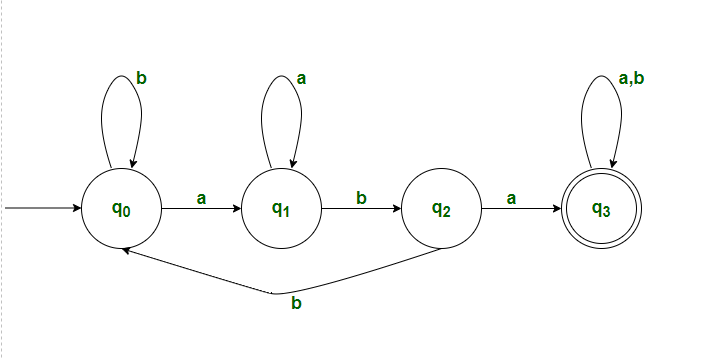Related Articles

# DFA accepting all strings over w ∈(a,b)* which contains “aba” as a substring

• Last Updated : 09 Dec, 2020

Given a binary string S, the task is to write a program for DFA Machine that accepts a set of all strings over w ∈ (a, b)* which contains “aba” as a substring.

Examples :

```Input-1 : ababa
Output : Accepted
Explanation : "ababa" consists "aba"

Input-2 : abbbb
Output : Not accepted
Explanation : "ababa" does not consist "aba"```

Approach : Below is the designed DFA Machine for the given problem. Construct a transition table for DFA states and analyze the transitions between each state. Below are the steps –Desired Language :

`L = {aba, baba, abab, aababbb.....}`

Explanation :

1. Firstly, there will be 4states.(say q0, q1, q2, q3) , with q0 being initial state and q3 being final state.
2. Initially we will be in q0 state, now we start reading the given string.
• When we read ‘b’, we will remain in the same state
• If we read ‘a’, then it transits to state q1.

3. Assuming that now we are in q1 state.

• When we read ‘a’, we will remain in the same state.
• If we read ‘b’, we will transits to state q2.

4. Assuming that now we are in q2 state.

• If we read ‘a’, it transits to state q3.
• If we read ‘b’, it transits to state q0.

5. Assuming we are in final state(q3)

• We remain in the same state, when we read ‘a’ or ‘b’.

6. All strings which are accepted by this DFA will have “aba” as its substring.

Transition Table :

Current stateFinal state
ab
q0q1q0
q1q1q2
q2q3q0
q3q3q3

Below is the implementation of the above approach –

## C++

 `// C++ program for the above approach``#include ``#include ``using` `namespace` `std;`` ` `// Function to check whether the given``// string is accepted by DFA or not``void` `checkValidDFA(string s)``{``    ``// Stores initial state of DFA``    ``int` `initial_state = 0;``    ``// Stores previous state of DFA``    ``int` `previous_state = initial_state;``    ``// Stores final state of DFA``    ``int` `final_state;``    ``// Iterate through the string``    ``for` `(``int` `i = 0; i < s.length(); i++) {``        ``// Checking for all combinations``        ``if` `((previous_state == 0 && s[i] == ``'a'``) || ``        ``(previous_state == 1 && s[i] == ``'a'``)) {``            ``final_state = 1;``        ``}``        ``if` `((previous_state == 0 && s[i] == ``'b'``) || ``        ``(previous_state == 2 && s[i] == ``'b'``)) {``            ``final_state = 0;``        ``}``        ``if` `(previous_state == 1 && s[i] == ``'b'``) {``            ``final_state = 2;``        ``}``        ``if` `((previous_state == 2 && s[i] == ``'a'``) || ``        ``(previous_state == 3)) {``            ``final_state = 3;``        ``}``        ``// Update the previous_state``        ``previous_state = final_state;``    ``}``    ``// If final state is reached``    ``if` `(final_state == 3) {``        ``cout << ``"Accepted"` `<< endl;``    ``}``    ``// Otherwise``    ``else` `{``        ``cout << ``"Not Accepted"` `<< endl;``    ``}``}`` ` `// Driver Code``int` `main()``{``    ``// Given string``    ``string s = ``"ababa"``;``    ``// Function Call``    ``checkValidDFA(s);``}`

## C

 `// C++ program for the above approach ``#include ``#include ``   ` `// Function to check whether the given ``// string is accepted by DFA or not``void` `checkValidDFA(``char` `s[] ) ``{``    ``// Stores initial state of DFA``    ``int` `initial_state = 0;``    ``// Stores previous state of DFA``    ``int` `previous_state = initial_state; ``    ``// Stores final state of DFA ``    ``int` `final_state;``    ``// Iterate through the string ``    ``for``(``int` `i = 0; i < ``strlen``(s); i++)``    ``{``      ``// Checking for all combinations     ``      ``if``((previous_state == 0 && s[i] == ``'a'``) || ``      ``(previous_state == 1 && s[i] == ``'a'``))``      ``{``          ``final_state = 1;``      ``}``      ``if``((previous_state == 0 && s[i] == ``'b'``) || ``      ``(previous_state == 2 && s[i] == ``'b'``))``      ``{``          ``final_state = 0;``      ``}``      ``if``(previous_state == 1 && s[i] == ``'b'``)``      ``{``          ``final_state = 2;``      ``}``      ``if``((previous_state == 2 && s[i] == ``'a'``) || ``      ``(previous_state == 3))``      ``{``          ``final_state = 3;``      ``}``        ``// Update the previous_state``        ``previous_state = final_state;``    ``}``    ``// If final state is reached``    ``if``(final_state == 3)``    ``{``        ``printf``(``"Accepted"``);``    ``}``    ``// Otherwise ``    ``else``    ``{``        ``printf``(``"Not Accepted"``);``    ``} ``}`` ` `// Driver Code``int` `main()``{``    ``// Given string``    ``char` `s[] = ``"ababa"``;``     ` `    ``// Function Call ``    ``checkValidDFA(s);``}`

Output :

`Accepted`

Time Complexity : O(N)
Auxiliary Space : O(1)

We provide nothing but the best curated videos and practice problems for our students. Check out the C Foundation Course and master the C language from basic to advanced level. Wait no more, start learning today!

My Personal Notes arrow_drop_up